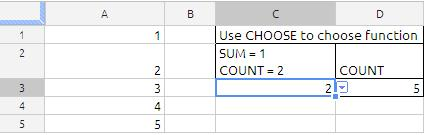We are looking into some more Weekday formula in addition to my earlier blog post about Weekday function.

In this example we are identifying  the first and last weekdays using the Weekday, Month, Date & MOD function in Google Docs Speadsheets.

The Chosen date is in cell B1,

the link to my earlier Weekday function

## WEEKDAY

WEEKDAY
The WEEKDAY function returns a number that is the day of the week for a given date.

WEEKDAY(number, type)

number:  The date the function should use. date is a date/time value. The time portion
is ignored by this function.

type: An optional value that specifies how days are numbered.

Sunday is 1 (1 or omitted):  Sunday is the first day (day 1) of the week and Saturday
is day 7.
Monday is 1 (2):  Monday is the first day (day 1) of the week and Sunday is day 7.
Monday is 0 (3):  Monday is the first day (day 0) of the week and Sunday is day 6.

Examples
=WEEKDAY(“2-Feb-2012”, 1) returns 5  (Thursday, the fifth day if you start counting Sunday as day 1).
=WEEKDAY(“2-Feb-2012”) returns the same value as the preceding example (numbering scheme 1 is used if no number-scheme argument is specified).

=WEEKDAY(“2-Feb-2012”,2) returns 4 (Thursday, the Fourth day if you start counting Monday as day 1).
=WEEKDAY(”2-Feb-2012”, 3) returns 3 (Thursday, day number 3 if you start counting Monday as day 0).

## CHOOSE

The syntax of the function Choose is as follow
CHOOSE(index, value1, … value30)

Uses an index to return a value from a list of up to 30 values. Index is a reference or number between 1 and 30 indicating which value is to be taken from the list. Value1, … value30 is the list of values entered as a reference to a cell or as individual values.

CHOOSE can return a value or a range from a list of 1 to 30 arguments.

When the “lookup_value” / index_number is whole number between 1 and 30, CHOOSE can be a good lookup tool

If index_num is 1, then value1 is used …
If index_num is 2, then value2 is used

value can be a number, or a formula/function, “text” (in quotes), a range, cell references, or defined names

To find next friday from any given day we can use Choose function
Suppose A1 hold the 1/1/2012 (mm/dd/yyyy)
the following formula will give the next friday

=CHOOSE(WEEKDAY(A1),A1+5,A1+4,A1+3,A1+2,A1+1,A1,A1+6,A1+7)

if we have data down as belowThe formula in cell D2 is
=CHOOSE(C3,”SUM”,”COUNT”)
if the cell C3 is 1 we will get SUM and if cell C3 is 2 we will get COUNT

The formula in cell D3 is
=CHOOSE(C3,SUM(A1:A5),COUNT(A1:A5))
In the example shown above the cell C3 is 2 so we will get the count of cells from A1 TO  A5
if the cell value in C3 is 1 we will get the SUM of cells from A1 to A5